Synthesis of Calcium Carbonate Experiment Lab report

Experiment – Synthesis of Calcium Carbonate

Directions: To complete the experiment, read the laboratory experiment in the manual, watch the video below, and complete the data table below.

The experimental report must be submitted to the appropriate Blackboard assignment folder.

Reading Assignment: Synthesis of Calcium Carbonate

 Experimental Videos: Synthesis of Calcium Carbonate https://youtu.be/kKNp0HVgmjI

Simulated Data:

Complete the data table. Remember Significant figures

 Molarity of sodium carbonate (mol/L) 0.110 Molarity of calcium chloride (mol/L) 0.100 Sodium Carbonate Solution Trial 1 Trial 2 Trial 3 Volume (mL) 50.0 50.0 50.0 Moles (mol) Calcium Chloride Solution Trial 1 Trial 2 Trial 3 Volume (mL) 50.0 50.0 50.0 Moles (mol) Solid Formed Trial 1 Trial 2 Trial 3 Mass of watch glass and filter paper (g) 56.665 61.023 38.607 Mass of precipitate, watch glass and filter paper (g) 56.986 61.315 38.961 Mass of precipitate (g) Actual moles of precipitate (mol) Theoretical moles of product formed (mol) Percent Yield Average Percent Yield Standard Deviation Percent Yield RSD of Percent Yield (%)

Post Lab Questions

1. Answer questions 1, 2, and 3 from the lab manual.

Synthesis of Calcium Carbonate

Introduction

A metathesis or double displacement (also referred to as double replacement) reaction is a reaction in which two compounds exchange ions to form two new compounds.

# AB + CD -4 AD + CB

A double displacement reaction usually occurs in solution and one of the products formed will be a solid and will precipitate out thereby driving the reaction forward. In other words, the solid product formed will be insoluble in solution. That is (assuming monovalent ions):

A(aq) + B(aq) + C(aq) + Döaq) Ataq) + DÜaq) + CB(s)

which is generally referred to as an ionic equation. In this simplified example, both A (aq) and D(aq) are present on both sides of the reaction and thus are spectator ions — they do NOT participate in the chemical reaction. Typically, the ionic equation will be further simplified to the net ionic equation where only the reacting species are shown: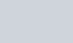+ ctaq) CB(s)

For example, if a solution of sodium• chloride (table salt) is mixed with silver nitrate, a white solid will form which is silver chloride. The molecular equation is:

NaCl(aq) + AgN03(aq) NaN03(aq) + AgClcs)

whereas the full ionic equation is: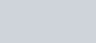+ CIGq) + Ageq) + –9 + + AgC1(s)

As both the sodium and nitrate ions remain in solution, the net ionic equation is: Cl(aq) + Agtaq) AgCl(s)

The amount of product(s) formed will be determined by the limiting reactant (also referred to as limiting reagent). The limiting reactant will be completely consumed during the reaction. The reactant that remains after the limiting reactant is consumed is referred to as the excess reactant (excess reagent). The excess reactant remains because there is nothing with which it can react.

For example, if you have ten (10) bicycle frames and twenty-three (23) bicycle tires, you can assemble only ten (10) complete bicycles. Since each complete bicycle used two (2) tires, a total of twenty (20) tires will be used and three (3) tires will be left over. In this example, the number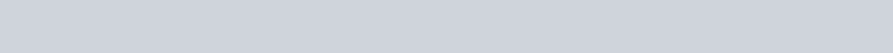This document was prepared by D. Finneran, J. Tierney.

62

There are multiple ways to identify the limiting reactant. One reactant (A) is chosen, and the bdanced chemical equation is used to determine the amount of the other reactant (B) necessary to react with A. If the amount Of B actually present exceeds the amount required, then B is in excess and A is the limiting reagent. If the amount of B present is less than required, then B is the limiting reactant. For example, if 119.3 grams of sodium chloride are reacted with 240.7 grams of silver nifrate, the limiting reactant can be determined:

1 mole NaC1 1 mole AgN03 169.87 gAgN03 119.3 g NaC1 x

58.44 g NaC1 1 mole AgN03 = 346.8 g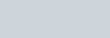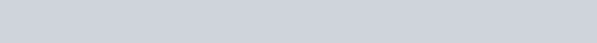1

mole

NaC1

As the amount of silver nitate (240.7 g) is less than required (346.8 g) to fully consume the sodium chloride present, silver nitrate is limiting and sodium chloride is in excess. Likewise, the amount of sodium chloride (119.3 g) exceeds the amount required (82.81 g) to fully consume the silver nitrate indicating that sodium chloride is present in excess and silver nitrate is limiting:

240.7 gAgN03 x 169.87 1 mole gAgN03 AgN03 1 1 mole mole AgN03 NaCl 58.44 g NaC1NaCl = 82.81 g NaC1Otherwise, the balanced chemical equation is used to calculate the amount of one product which can be formed from each reactant in the amount present. This method can be extended to any number of reactants more easily than the first method. Whichever reactant forms the least amount of a given product is the limiting reactant:

1 mole NaC1 1 mole AgC1 143.32119.3 g NaC1 x 58.44 1 mole NaC1 1 mole AgCl = 292.6 gAgC1

1 mole AgN03 1 mole AgCl 143.32 g AgC1

240.7 g AgN03 X gAgN03 x 1 mole AgN03 x 1 mole AgCl = 203.1 g AgC1 169.87

Since the full reaction of the silver nitrate produces a lesser amount of product (silver chloride), the silver nitrate is limiting.

The limiting reagent must be identified so that theoretical and percentage yield may be calculated. theoretical yield is defined as the amount of product obtained when the limiting reagent reacts completely taking into account the stoichiometry of the balanced reaction. fractional yield or relative yield is used as a measure of the effectiveness of the procedure. The fractional yield is simply calculated by dividing the actual amount of product by the theoretical amount of product.

Actual Yield

Fractional Yield Theoretical YieldThe percentage yield is simply the fractional yield multiplied by 100%.

Actual Yield x 100%

Percentage Yield Theoretical Yield document was prepared by D. Finneran, J. Tierney.

63

If 185.4 grams of silver chloride were collected in the example above, then the fractional yield would be:

Actual Yield 185.4 g AgC1 = 0.9219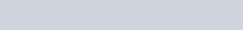Fractional Yield =

Theoretical Yield 203.1 g AgCl or a percent yield of 92.19%.

Safety Precautions

You are using common materials here that do not pose a known health risk. As with all laboratory experiments, wear safety glasses at all times. All solutions may be safely discarded down the drain. ‘Ihe solid and filter paper may be discarded in the regular trash.

Procedure — Obtaining the Data

You should know how to use the analytical balance. If you are unsure, please ask your instructor for assistance.

1. Obtain a bottle of 0.110 M sodium carbonate solution and a bottle of 0.100 M calcium chloride solution.
2. Add approximately 50.0 mL of 0.110 M sodium carbonate solution to a clean 250 mL beaker. Record the volume actually added.
3. Add approximately 50.0 mL of 0.100 M calcium chloride solution to a DIFFERENT clean beaker. Record the volume actually added.
4. Using a hotplate, gently warm both beakers for five (5) to ten (10) minutes. You do NOT need to boil the solutions.
5. After the solutions are warmed — remove both from the hot plate using gloves or beaker tongs. Remember — the glass is HOT.
6. Pour the contents of one beaker into the other. The solutions are warmed so that when mixed, the solid particles formed will increase in size. The warmer the solutions are, the faster fflis process will occur. If you attempt to filter too quickly, the solid precipitate particles are small enough that they will pass through the pores of the filter.
7. Allow the mixture to cool down to room temperature. Do NOT place in ice.
8. While the mixture is cooling, set up a vacuum filter apparatus.
9. Label a piece of filter paper with your initials.
10. Place the filter paper on a watch glass and record the mass.
11. Vacuum filter the mixture once it reaches room temperature.
12. Place filter paper on watch glass and place in oven.
13. Dry for a minimum often (10) minutes to evaporate any remaining water.
14. OPTIONAL – YOUR INSTRUCTOR WILL NFORM YOU IF IT IS NECESSARY TO CARRY OUT THESE STEPS. IF SO, YOU WILL NEED To MAKE YOUR OWN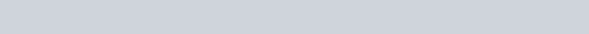a. Record the mass of a clean, dry beaker.

the filfrate into one of this clean, dry beaker using ab.

This document was prepared by D. Finneran, J. Tierney.

64

1. Place the beakers on a hot plate to boil and evaporate the water. CAUTION – As the solution level decreases, the risk of splattering increases.
2. Once the water has completely evaporated, allow the beaker to cool to room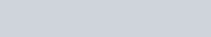1. weigh the beaker and the mass of the solid(s) and beaker. Determine the mass of solid(s) by difference.
2. Discard the solid(s) in the regular trash or rinse down the sink with water.
3. Remove from the oven and allow to cool to room temperature. Determine the mass of the watch glass, filter paper, and solid.
4. Determine the mass of the solid by difference.
5. Determine the moles of the solid (hint — write out the balanced equation first). If you carried out step 14, determine the moles of the solid(s) in the beaker as well.
6. Identify the limiting reactant.
7. Determine the theoretical and percent yield.
8. Repeat the above process two more times.
9. Determine your average percent yield, standard deviation, and relative standard deviation of percent yield. Provide this information to the class by posting on the Course Management system (ANGEL, Blackboard, etc.) or as otherwise instructed within 48 hours of completion of the laboratory experiment.

J. Tierney. s Finneran,

Synthesis of Calcium Carbonate Data Sheet

Name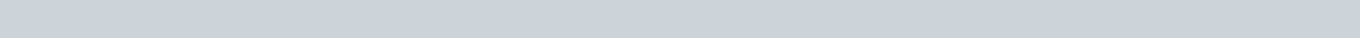Name

Partner’s

Molarity of the sodiun carbonate used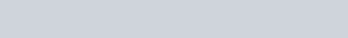Molarity of the calcium chloride solution used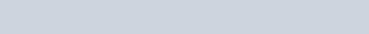Trial 2 Sodium Carbonate Volume of sodium carbonate soluEon used (n-L) Moles of sodium carbonate used (mol) Calcium Chloride Solution Volume of the calciun cåoride sohÆon used (rnL) Moles of calciu-n chloride used (mol) Solid Formed Mass ofwatch glass and filter paper @ Mass ofptecipitate, watch gass and Nte: paper@ Mass ofprecipitate @ Actual moles of precipitate (mol) TheorÆca1 moles ofproduct formed (mol) Percent Yield

Overall Results

 Average Percent Yield Standard Percent Yield RSD ofPercent Yield C/o) Class Average Percent Yield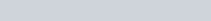was prepared by D. Finneran,

General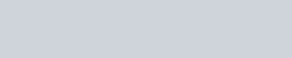This document J. Tiemey.

66

Synthesis of Calcium Carbonate Post-Lab Questions

Name

please type your answers to the following questions on as many pages as needed. Non-typed answers not be accepted.

1. Write out the balanced reaction.
2. Write out the full ionic equation.
3. Write out the net ionic equaüon.
4. How does your average percent yield compare to the class? Explain.
5. How does your precision compare to the class? Explain.
6. Have you used either one of the products in a previous experiment? Which one(s)? Which experiment(s)?
7. Could ammonium carbonate solution have been used in lieu of sodium carbonate solution? If assuming the same concentrations as used in this experiment, what would the limiting reactant be? Hint — think about solubility.
8.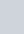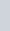Could aluminum carbonate solution have been used in lieu of sodium carbonate solution? If so, assuming the same concentrations as used in this experiment, what would the limiting reactant be? Hint — think about solubility.

9, Could calcium nitride solution have been used in lieu of calcium chloride solution? If so,assuming the same concentrations as used in this experiment, what woüld the limiting reactant be? Hint — think about solubility.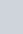Could calcium bromide solution have been used in lieu of calcium chloride solution? If so, assuming the same concentrafions as used in this experiment, what would the limidng reactant be? Hint think about solubility.

I I • If you carried out the optional step, which component was easier to recover and why?

12• If you carried out the optional step, how do the percent yields compare?

IS document was prepared by D. Finneran, J, Tierney.

67